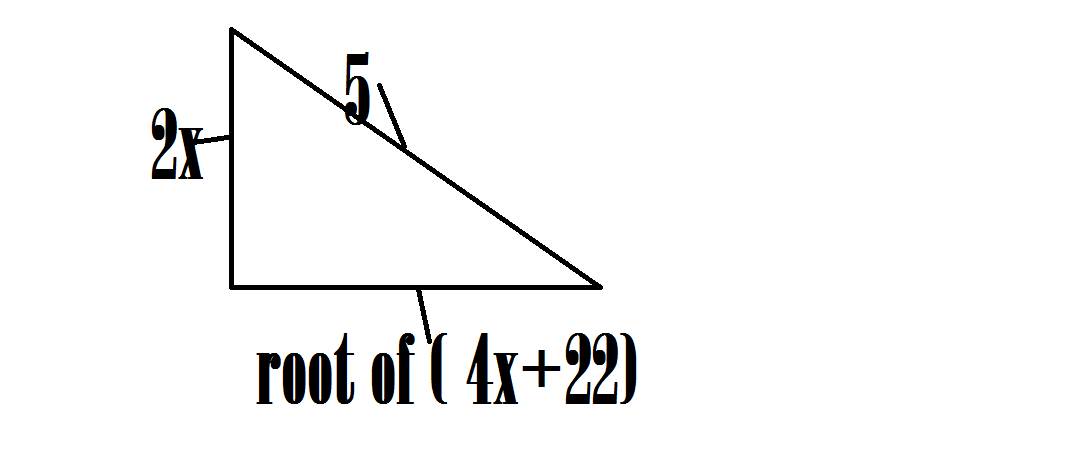A geometry problem by Aareyan Manzoor

Geometry Level 2Given that the triangle above is a right-angle triangle, find $x$ and round your answer to three decimal places.

×